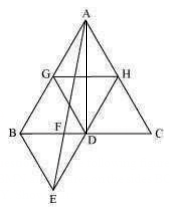Guru

# In Fig.9.33, ABC and BDE are two equilateral triangles such that D is the mid-point of BC. If AE intersects BC at F, show that:(ii) ar (BDE) = ½ ar (BAE) Q.5(2)

• 0

Please guide me the best way for solving the question of class 9th math of Areas of Parallelograms and Triangles chapter of exercise 9.4 of math of question no.5(2)  What is the best way for solving this question, please guide me In Fig.9.33, ABC and BDE are two equilateral triangles such that D is the mid-point of BC. If AE intersects BC at F, show that:(ii) ar (BDE) = ½ ar (BAE)

Share

1.ar(ΔBDE) = ar(ΔAED) (Common base DE and DE||AB)

ar(ΔBDE)−ar(ΔFED) = ar(ΔAED)−ar (ΔFED)

ar(ΔBEF) = ar(ΔAFD) …(i)

Now,

ar(ΔABD) = ar(ΔABF)+ar(ΔAFD)

ar(ΔABD) = ar(ΔABF)+ar(ΔBEF) [From equation (i)]

ar(ΔABD) = ar(ΔABE) …(ii)

AD is the median of ΔABC.

ar(ΔABD) = ½ ar (ΔABC)

= (4/2) ar (ΔBDE)

= 2 ar (ΔBDE)…(iii)

From (ii) and (iii), we obtain

2 ar (ΔBDE) = ar (ΔABE)

ar (BDE) = ½ ar (BAE)

Hence proved

• 0